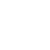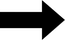home

# Kauffman's NK Model

## Choose the number of genes (N) and number of interactions per gene (K)...

Stuart Kauffman's NK model let's us explore correlated, tunably-rugged fitness landscapes. Roughly, N is the number of genes, and K is the number of other genes that each gene interacts with. If you'd like to learn more about it you can read here: http://en.wikipedia.org/wiki/NK_model
N (1-100):
K (0-12):

Change Parameters### State of 100 binary traitsFitness Score
SHOW ACCEPTABLE MUTATIONS

The NK model does an excellent job at exhibiting how interactions affect dynamics on adaptive landscapes. The more interactions that take place in a system, the more "rugged" the landscape is in regards to fitness - with more interactions you get more sub-optimal peaks. In this extremely simplified model, we can tune this ruggedness, by changing the number of squares (which could also be called traits, or alleles, or parts) that each square interacts with. Each square can take on only one of two states (colors), and its fitness contribution depends on its state and the state of its K interactors. You can change K above and click submit to load up the system (you can also change N, the total number of squares). Then you can click above to change the color of each square and see how fitness responds, or you can simulate using a simple algorithm to increase fitness on the right. If you click the button above, the acceptable mutations (beneficial or nearly neutral) are shown with a green border. You can also click on any of the question mark blocks for more information about that part of the model.

#### The State

The squares above can each have one of two possible states - red or grey. You can click on them to switch between the two states. The state of the entire system is defined by the state of each individual square. In analogy with biology, these squares could be thought of as genes with two different alleles, or as nucleotides in a DNA sequence, but with only two possible bases rather than four. In this model each of these "genes" interacts with K other genes, chosen at random from all of the squares. The spatial layout of the squares above has no meaning within the model - it's just the easiest way to arrange a bunch of squares! (I know it looks like some spatial model or a cellular automaton - which are very cool things, but different from this). The number of possible states is 2 raised to the power of the number of squares - this number grows very large as the number of squares goes up - for example for a set of 25 squares, there are over 30 million possible states.

#### The Fitness Mapping

The fitness mapping, or fitness function, describes how we assign fitness values to each of the possible states for the grid of squares. For this model, we assign to each of the N squares K other squares as interactors. Now we assign a random fitness contribution (between 0.0 and 1.0) to each of the possible combinations of the state (color) of the square in question and the state of its K interactors. The total fitness score is the average of all of these contributions. If K=0 each square has only two possible fitness contributions, one for red and one for grey. In the landscape metaphor, where fitness is the analog of elevation, we call this a Mt. Fuji landscape, with one towering smooth peak. If K=1 each square has 4 possible fitness contributions, assigned randomly, based on its state and the state of the other square that affects it. This landscape is more rugged than the K=0 case. In general, each square will have 2^(K+1) possible fitness contributions, since that is the number of possible states for the square and its K interactors. If K=N-1, every click changes the contribution of every other square (they all interact), so we a completely random, extremely rugged landscape. In between, we can produce landscapes that are correlated but rugged. The reason behind this tunable-ruggedness is that changes in one gene have different effects depending on the alleles of other genes. This is precisely how we think of epistasis in genetics, and it is certainly a reality of life and of other complex systems.

#### Simulating Change

This simulation runs a very simple algorithm. Here it is:

1) Randomly choose a trait to change
2) If the new state results in a higher fitness, keep the change
OR if the new state results in a fitness within the "nearly neutral" range and the box is checked, keep the change
3) If not, reject the change and revert to the old state (this still counts as a "step" on the graph)

This is clearly not an accurate representation of how biological evolution occurs, but it does capture some of the dynamics of how change can happen in systems with many interacting parts. So how accurate is this algorithm? What would be a proper way of simulating evolution or mathematically describing how a population or species changes over time? I'm working on a page going into these questions in more detail, thinking about population genetics and describing evolutionary change from the bottom-up or top-down. I will link to that here when I finish it

#### Simulate ChangeAccept (Nearly) Neutral Mutations?
Range to Consider Nearly Nuetral:

Update
options
GO
STOP

The simulation will stop automatically every 800 steps
NEW RUN
RESET